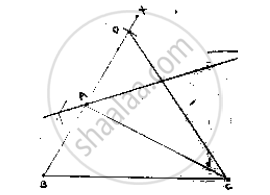# Using Ruler and Compasses Only, Construct a δAbc, Given Base Bc = 7cm, ∠Abc = 60° and Ab + Ac = 12 Cm. - Mathematics

Using ruler and compasses only, construct a ΔABC, given base BC = 7cm, ∠ABC = 60° and AB + AC = 12 cm.

#### SolutionSteps of construction:
1. Draw a line segment BC of 7cm .
2. At B, draw an angle XBC of 60°
3. With center B and radius 1 2 , cm draw an arc which intersects BX at D.
4. Join DC
5. Draw the perpendicular bisector of DC which intersects BD at A.
6. Join AC
∴ΔABC is the required triangle.

Concept: Some Constructions of Triangles
Is there an error in this question or solution?

#### APPEARS IN

RD Sharma Mathematics for Class 9
Chapter 16 Constructions
Exercise 16.3 | Q 4 | Page 18

Share